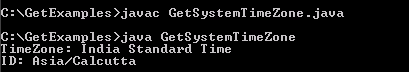Tutorials

# Java get System TimeZone

In this section, you will learn how to obtain the System's TimeZone. The Time Zone is basically the uniform standard time usually referred to as the local time.

In this section, you will learn how to obtain the System's TimeZone. The Time Zone is basically the uniform standard time usually referred to as the local time.

# Java get System TimeZone

In this section, you will learn how to obtain the System's TimeZone. The Time Zone is basically the uniform standard time usually referred to as the local time. We have used the instance of the class TimeZone in order to get the timeZone by using the method Calendar.getInstance().getTimeZone().

tz.getDisplayName()- This method returns the name of the time zone.

tz.getID()- This method returns the id of the time zone.

## Understand with Example

In this example, we want to display the System's Time zone.For this we consider a class GetSystemTimeZone,Inside the main method, we  use the instance of  class Time Zone to obtain the time zone of the specified region by calling  Calendar.getInstance( ).getTimeZone( ) method. The System.out.print ln is used to display the output by calling the following given below method-

1)getDisplayName( )-Provides you the name of time zone.

2)getID( )-  Provides you the id of time zone.

On Execution of the program code, The code returns you Time zone and ID.

Here is the code of GetSystemTimeZone.java

 ``` import java.util.*; public class GetSystemTimeZone {  public static void main(String[] args) {  TimeZone tz = Calendar.getInstance().getTimeZone();  System.out.println("TimeZone: "+tz.getDisplayName());  System.out.println("ID: "+tz.getID());   }```

Output will be displayed as: